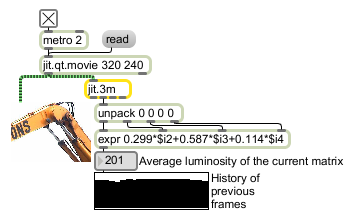# jit.3m

Report min/mean/max values

## Description

The jit.3m object reports the minimum, mean, and maximum values for each plane of a given input matrix as lists of size planecount , one value for each plane. The list of minimum values are sent out the left outlet of the object, the list of mean values are sent out the middle outlet, and the list of maximum values is sent out the right outlet.

## Matrix Operator

matrix inputs:1, matrix outputs:0

## Attributes

Name Type g/s Description
max atom (get) Displays the list of maximum values (per plane) calculated from the most recent input matrix.
mean atom (get) Displays the list of mean values (per plane) calculated from the most recent input matrix.
min atom (get) Displays the list of minimum values (per plane) calculated from the most recent input matrix.

## Examples